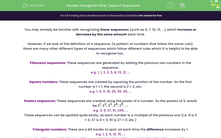# Recognise Other Types of Sequences

In this worksheet, students will recognise and define the types of sequences shown, as well as finding unknown terms by continuing sequences in the same pattern.Key stage:  KS 4

Year:  GCSE

GCSE Subjects:   Maths

GCSE Boards:   AQA, Eduqas, Pearson Edexcel, OCR,

Curriculum topic:   Algebra

Curriculum subtopic:   Sequences

Popular topics:   Square Numbers worksheets

Difficulty level:#### Worksheet Overview

You may already be familiar with recognising linear sequences (such as 4, 7, 10, 13, ...) which increase or decrease by the same amount each time.

However, if we look at the definition of a sequence, (a pattern of numbers that follow the same rule), there are many other different types of sequences which follow different rules which it is helpful to be able to recognise too.

Fibonacci sequences: These sequences are generated by adding the previous two numbers in the sequence.

e.g. 1, 1, 2, 3, 5, 8, 13, 21, ...

Square numbers: These sequences are created by squaring the position of the number. So the first number is 1 × 1, the second is 2 × 2, etc.

e.g. 1, 4, 9, 16, 25, 36, 49, ...

Powers sequences: These sequences are created using the power of a number. So the powers of 3, would be 31, 32, 33, 34, 35, ...:

e.g. 3, 9, 27, 81, 243, ...

These sequences can be spotted quite easily, as each number is a multiple of the previous one (i.e. 9 is 3 × 3; 27 is 9 × 3; 81 is 27 × 3; etc.)

Triangular numbers: These are a bit harder to spot, as each time the difference increases by 1.

e.g. 1, 3, 6, 10, 15, ...

1 to 3 = difference of 2; 3 to 6 = difference of 3; 6 to 10 = difference of 4; 10 to 15 = difference of 5, etc.

In this activity, we will recognise and define the types of sequences shown, as well as finding unknown terms by continuing sequences in the same pattern.

### What is EdPlace?

We're your National Curriculum aligned online education content provider helping each child succeed in English, maths and science from year 1 to GCSE. With an EdPlace account you’ll be able to track and measure progress, helping each child achieve their best. We build confidence and attainment by personalising each child’s learning at a level that suits them.

Get started#### Popular Maths topics

••••